# Inverse Gaussian Ornstein-Uhlenbeck Stochastic Clocks

In this pose, we consider an Ornstein-Uhlenbeck stochastic clock whose instantaneous rate of activity process has an inverse Gaussian stationary distribution. We use the previously obtained relationship between the cumulant generation function of the stationary distribution and that of the background driving Lévy process and show that the latter can be represented as the sum of an inverse Gaussian processes and a compound Poisson process with chi-squared distributed increments.

Related Posts

Construction via the Background Driving Lévy Process

We assume that the stationary distribution of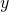is inverse Gaussian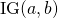with characteristic exponentThis is the distribution of the first hitting time of a drifted Brownian motionto the level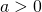, where; see e.g. Schoutens (2003). As shown in a previous post, the following relationship holdsThus,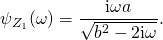As shown by Barndorff-Nielsen (1998) we can decompose the background driving Lévy process as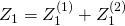whereandHere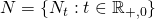is a Poisson process with arrival rate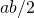and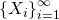is a sequence of independent identically distributed (i.i.d.) chi-squaredrandom variables with degree of freedom one; see also Schoutens (2003). We haveNext,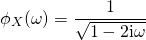and thus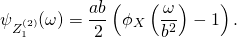Here, we used the general form of the characteristic function for compound Poisson processes. Summing the cumulant generating functions yieldsas claimed.

Integrated Time-Change

We again start from the previously obtained general solution for the characteristic function of the total activity process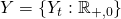,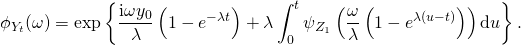We evaluate the two integrals corresponding toandseparately. The first one is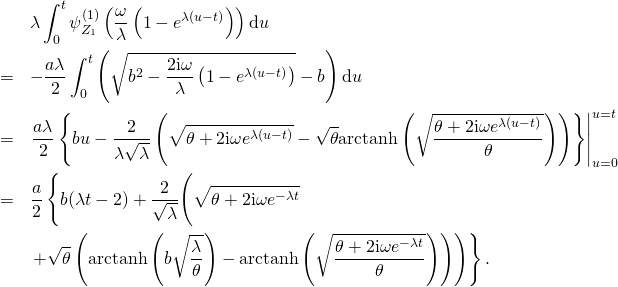Here, we defined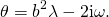The second one isCombining these two expressions and grouping terms yields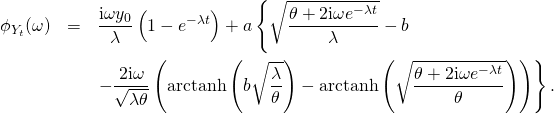This expression coincides with the one given in Schoutens (2003), who defines